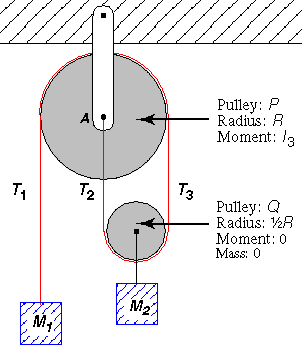*echo Pulley Problem

## Pulley ProblemA weightless and unstretchable string has one end fixed at the point A. The string passes under a movable pulley Q (of radius R/2 but negligible mass and moment of inertial) carrying a mass M2. Then the string passes over a fixed pulley P of moment of inertia I3, and finally the string is attached to mass M1 at its other end, as shown. The pulleys have frictionless axles but the string doesn't slip on their rims.
1. What should be the relation between M1 and M2 for static equilibrium?
M1/M2 =

2. When M1 moves down 1 meter, how far up does M2 move?
m

Now consider the acceleration of the system when the static equilibrium condition of part (a) above does not hold.

Answer the following in terms of M1 (M1), M2 (M2), I3 (I3), the tensions T1 (T1), T2 (T2), and T3 (T3) and g (g). For example, the answer to (c) is: M1*g-T1

3. What is the net downward force on M1?

4. What is the net counterclockwise torque on the fixed pulley P?

5. What is the net counterclockwise torque on the lower pulley Q?

6. What can you say about the relation between T2 and T3?
T3/T2 =

Why?

7. What is the net upward force on the M2 and pulley Q combination?

8. Proceed to solve for the acceleration of M1 in terms of the mass M2, moment of inertia I3, radius R, and g.